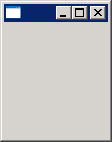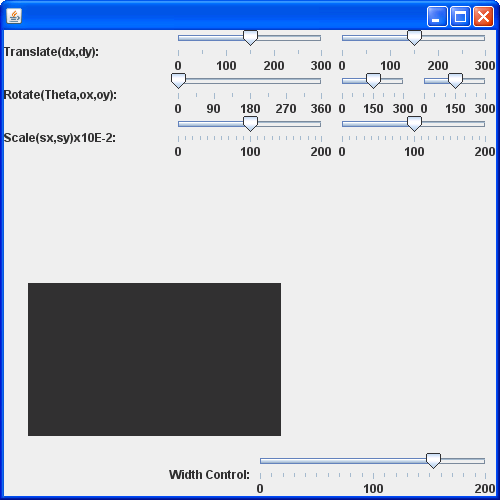# Draw Dashed : Line « 2D Graphics « Java Tutorial

```/*
Copyright (C) 2006 The Lobo Project

This library is free software; you can redistribute it and/or
modify it under the terms of the GNU Lesser General Public
version 2.1 of the License, or (at your option) any later version.

This library is distributed in the hope that it will be useful,
but WITHOUT ANY WARRANTY; without even the implied warranty of
MERCHANTABILITY or FITNESS FOR A PARTICULAR PURPOSE.  See the GNU
Lesser General Public License for more details.

You should have received a copy of the GNU Lesser General Public
License along with this library; if not, write to the Free Software
Foundation, Inc., 51 Franklin St, Fifth Floor, Boston, MA  02110-1301  USA

Contact info: lobochief@users.sourceforge.net
*/

import java.awt.*;

public static Frame getTopFrame() {
Frame[] frames = Frame.getFrames();
for(int i = 0; i < frames.length; i++) {
if(frames[i].getFocusOwner() != null) {
return frames[i];
}
}
if(frames.length > 0) {
return frames;
}
return null;
}

public static void drawDashed(Graphics g, int x1, int y1, int x2, int y2, int dashSize, int gapSize) {
if(x2 < x1) {
int temp = x1;
x1 = x2;
x2 = temp;
}
if(y2 < y1) {
int temp = y1;
y1 = y2;
y2 = temp;
}
int totalDash = dashSize + gapSize;
if(y1 == y2) {
int virtualStartX = (x1 / totalDash) * totalDash;
for(int x = virtualStartX; x < x2; x += totalDash) {
int topX = x + dashSize;
if(topX > x2) {
topX = x2;
}
int firstX = x;
if(firstX < x1) {
firstX = x1;
}
if(firstX < topX) {
g.drawLine(firstX, y1, topX, y1);
}
}
}
else if(x1 == x2) {
int virtualStartY = (y1 / totalDash) * totalDash;
for(int y = virtualStartY; y < y2; y += totalDash) {
int topY = y + dashSize;
if(topY > y2) {
topY = y2;
}
int firstY = y;
if(firstY < y1) {
firstY = y1;
}
if(firstY < topY) {
g.drawLine(x1, firstY, x1, topY);
}
}
}
else {
// Not supported
g.drawLine(x1, y1, x2, y2);
}
}
}
```
 16.12.Line 16.12.1. Draw a line16.12.2. draw Line from (10, 15) to (100, 115) 16.12.3. Line2D.Float16.12.4. Draw a point: use a drawLine() method 16.12.5. A line is drawn using two points 16.12.6. Dash style line 16.12.7. Line dashes style 2 16.12.8. Lines Dashes style 3 16.12.9. Line Dash Style 4 16.12.10. Draw Dashed 16.12.11. Compares two lines are returns true if they are equal or both null. 16.12.12. Creates a region surrounding a line segment by 'widening' the line segment.